# Two phase flow

After the code validation in monophase flow case, we decided to add an additional phase to the solver. Then we developed a code that we called impesFoam. In the code, we resolved the conservation equations of the two phases to obtain the saturation and the the pressure field. We called the phase corresponding to air phase a and the phase that corresponds to the liquid (oil or water) phase b.

We used in all our studies the same geometry as the geometry that has been used in the previous parts which corresponds to a simple square, however the well size will be modified in certain cases.

First of all we resolved low and high values of saturation problems, after that we added to the developed solver the capillary pressure  and finally we did several simulations to study different regimes.

# Models used

In the  developed solver we resolve the  conservation equations to obtain the saturation field and the pressure field.

In general case of an isothermal flow we have to calculate in each point of the porous media and as function of time :

• The pressure in each fluid $P_a$ and $P_{b}$
• The saturation in each fluid $S_a$ and $S_{b}$
• The velocity in each fluid $U_a$ and $U_{b}$

• The density in each fluid is $\rho_a$ and $\rho_{b}$, we supposed that the densities of fluids are equal and we call them  $\rho$ .
•  The viscosity in each fluid are $\mu_a$ and $\mu_{b}$

Then we have the following equations :

Darcy law :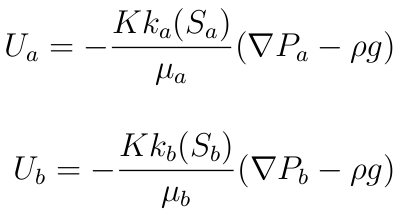State equations :

We assume that the flow  is isothermal, moreover the density and the viscosity are supposed constant.

Continuity equations :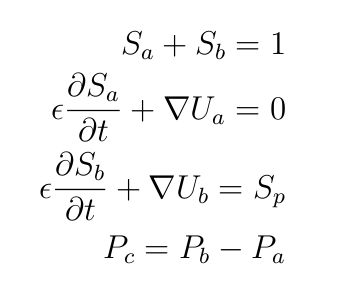# Resolution method

From the previous equations we obtained equation on the pressure :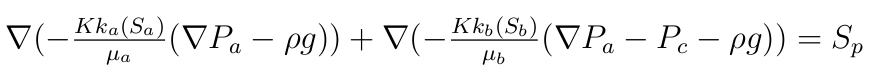The pressure in the phase b is obtained by subtraction.

And we resolved the equation of the saturation of the phase b only :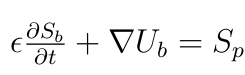The saturation of the phase a is obtained by subtraction.

In the developed two-phase flow  solver we used a method called IMPES (Implicit Pressure Explicit Saturation). In this method the equation on the pressure is resolved in an implicit manner. Moreover the saturation of the liquid phase is resolved explicitly.

Then we resolve the equations of pressure and saturation separately. The positive point of this method is that we gain time of calculation, however the IMPES method could create in certain configurations instabilities during the saturation transport.

# Negative saturation problem

In some cases and due to the instabilities created by the IMPES Method we observe that after certain time of extraction of liquid, the saturation could be negative or superior to one which is incompatible with our definition of the saturation.

Then we added a criteria on the saturation to avoid having negative saturation. The criteria stops the well production if there is a negative saturation in one or several cells of the well.

To resolve saturation superior to one problem, one of the solution  is to decrease gradually the source term (flow rate) when the saturation goes below certain values fixed by the operator. This could help to delay the apparition of saturation superior to one.

# Simulation of different regimes (without capillary pressure)

The aim of this part is to highlight the existence of different regimes that depend on the source term ( flow rate in the well) , on the fluid and the ground properties.

We will launch different simulations for different rate flow values to detect those regimes.

To study the existence of different regime we will plot the saturation for different times and for different flow rates values.

The domain dimension for all the following simulations is 10m*10m.

# Oil production : low flow rate regime

We have a mesh 40*40 cells and the a well of 20cm*50cm.

In the following simulation when the oil saturation goes under the value 0.1 we stop the well production.

we had the following parameters :

phase a :

•  Viscosity $\mu_a=1.17\ kg/(m.s)$
• density $\rho_a=1.85 \ 10^{-5} kg/m^3$

phase b :

•  Viscosity $\mu_b=1 \ 10^{-1} kg/(m.s)$
• density $\rho_b=0.88 \ 10^3 Kg/m^3$

The initial flow rate qwell was fixed to :

•  $qwell=5 \ 10^{-5} m^3/s$

Moreover we consider that we have an isotropic domain and we fixed the permeability to :

•  $K=1 \ 10^{-9}m^2$

Here we can see the representation of the domain :

Well and domain representationThis the initial saturation that  has been taken :

Initial oil staurationSaturation in oil time=63500 sSaturation in oil time=203500 sAs the oil considered in those simulations is very viscous ( $\mu_b=1 \ 10^{-1} kg/(m.s)$ ) this decreases the mobility and it is not possible to pump with high value of the rate flow. Indeed pumping very viscous liquid with high rate flow results in a creation of a "hole in the domain" and the introduction of air in the well which disturb significantly the production. The rate flow adapted to this liquid/configuration should be around $qwell=1 \ 10^{-5} m^3/s$}.

Example of " hole" in the saturation created in the case of $qwell=5 10^{-5} \ m^3/s$ and $K=10^{-10} m^2$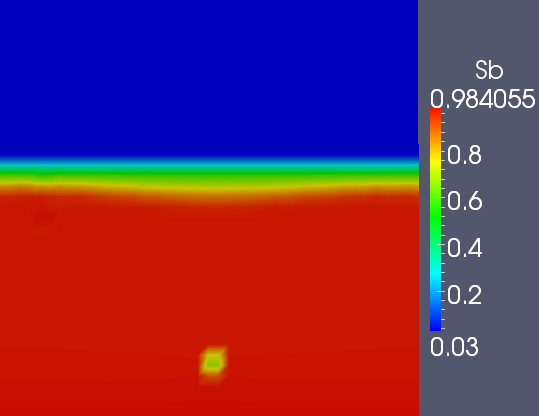Indeed we can see on the previous figure that with very viscous fluid and very low permeability we cannot pump with very high flow rates.

# Water production : high flow rate regime

We have a mesh 120*120 cells and the a well of 50cm*200cm.

We took in this part of fluid that have properties similar to the water properties but less dense than water.

Here we can see the representation of the domain :

Well and domain representationThe initial saturation will have the form of this :

Initial saturation in waterWe implemented a first simulation with the following parameters

phase a :

• Viscosity $\mu_a=1.17 \ kg/(m.s)$
•  density $\rho_a=1.85 \ 10^{-5} kg/m^3$

phase b :

• Viscosity $\mu_b=1 \ 10^{-3} kg/(m.s)$
• density $\rho_b=0.88 \ 10 ^3 Kg/m^3$

The initial flow rate qwell was fixed to :

•  $qwell=1 \ 10^{-2} m^3/s$

Moreover we consider that we have an isotropic domain and we set the permeability to :

•  $K=1 \ 10^{-10}m^2$

In this part when the saturation in water goes below 0.5, the initial flow rate is gradually decreased. Furthermore  when the water saturation goes below the value 0.1 we stop the well production.

Saturation in water time=300 sSaturation in water time=350 sSaturation in water time=650 sSaturation in water time=4000 s# Capillary pressure influence

As it is known, in multiphase flow it could exist a  difference of pressure between phases present in the porous media. The difference of pressure is called the capillary pressure and is due to the existence of surface tension between the phases involved.

When we take into account the capillary pressure, we have a gradient of this pressure added to the conservation equations.

# Capillary pressure modeling

In the literature  exists several empirical formula of capillary pressure present in a porous media.

To understand the effect of the capillary pressure on the saturation, we did our simulation with the following simple formula as a first approach :

$P_c=C(S_e)^{-\alpha}$

where :

•  $P_c$ is the capillary pressure
•  $C$ and $\alpha$ are constant
•  $S_e$ is the effective saturation in liquid.

Se is defined by :• where $S_{min}$ and $S_{max}$ are the minimal and the maximal saturation of the porous media.

# Simulations

We did all the  simulations in this part with the same parameters as in 3.3.1 and by taking into accounting the presence of a capillary pressure with $\alpha=0.2$ and $C=10.10^3 Pa$.

We are going to compare the oil saturation over a vertical line from the domain with capillary pressure and without capillary pressure.

Plot line :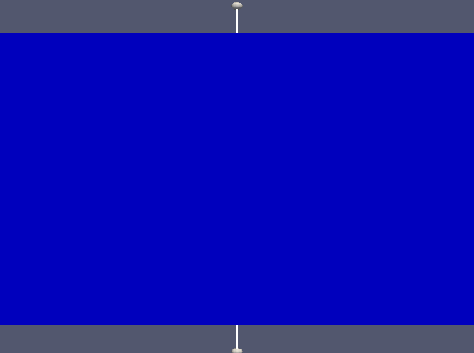# Simulations with capillary pressure and qwell=0

In order to observe the effect of the capillary pressure on the saturation we set the source term equal to 0.

Oil saturation t=0 sOil saturation t=130000 sWe observe that saturation is increased in the boundary region between oil and air. Indeed the water goes up due to the capillary pressure, after certain time the water stop going up due the balance by gravity

# Simulations with capillary pressure with pumping

We compare the influence the case with capillary and the case without capillary pressure.

Saturation without $P_c$, time=100000 sSaturation with $P_c$,  time=100000 sWe observe that the capillary pressure allows the oil to go up and help to the pumping .
Moreover we know that pumping create a lack of oil which increases the influence of the capillary pressure.

# Two phase flow conclusion

We succeeded to implement the solver and resolve problems such as the problem of negative saturation.

However some cases with high  rate flow production are not yet stabilize and leads to a saturation superior to one which causes a divergence of the solver.

The study of viscous flow and in domains with small permeability  has shown that we  have a limit on the rate flow of production. Indeed when this limit is reached a "hole" is created in the liquid saturation field.